Vibroengineering PROCEDIA

Published: 05 November 2020

# Investigation on the wake of a single-box girder in sinusoidal oscillating flow

Zhao Xiao1
Liangliang Zhang2
Zhijun Ni3
1, 2, 3School of Civil Engineering, Chongqing University, Chongqing, China
Corresponding Author:
Liangliang Zhang
Views 22

#### Abstract

The wake of a single-box girder in sinusoidal oscillating flow is studied by three-dimensional large-eddy simulations (3D LES). The validation of numerical simulation is verified by active-controlled wind tunnel experiments. The mean and fluctuating velocity, as well as the vortex shedding distance in the sinusoidal oscillating flow are discussed. The fluctuating velocity near the wake increases with the increasing oscillation amplitude, however it is distribution period independent of oscillation amplitude. The vortex shedding distance decreases as the oscillation frequency increases, meanwhile, the oscillation amplitude has no effect on it.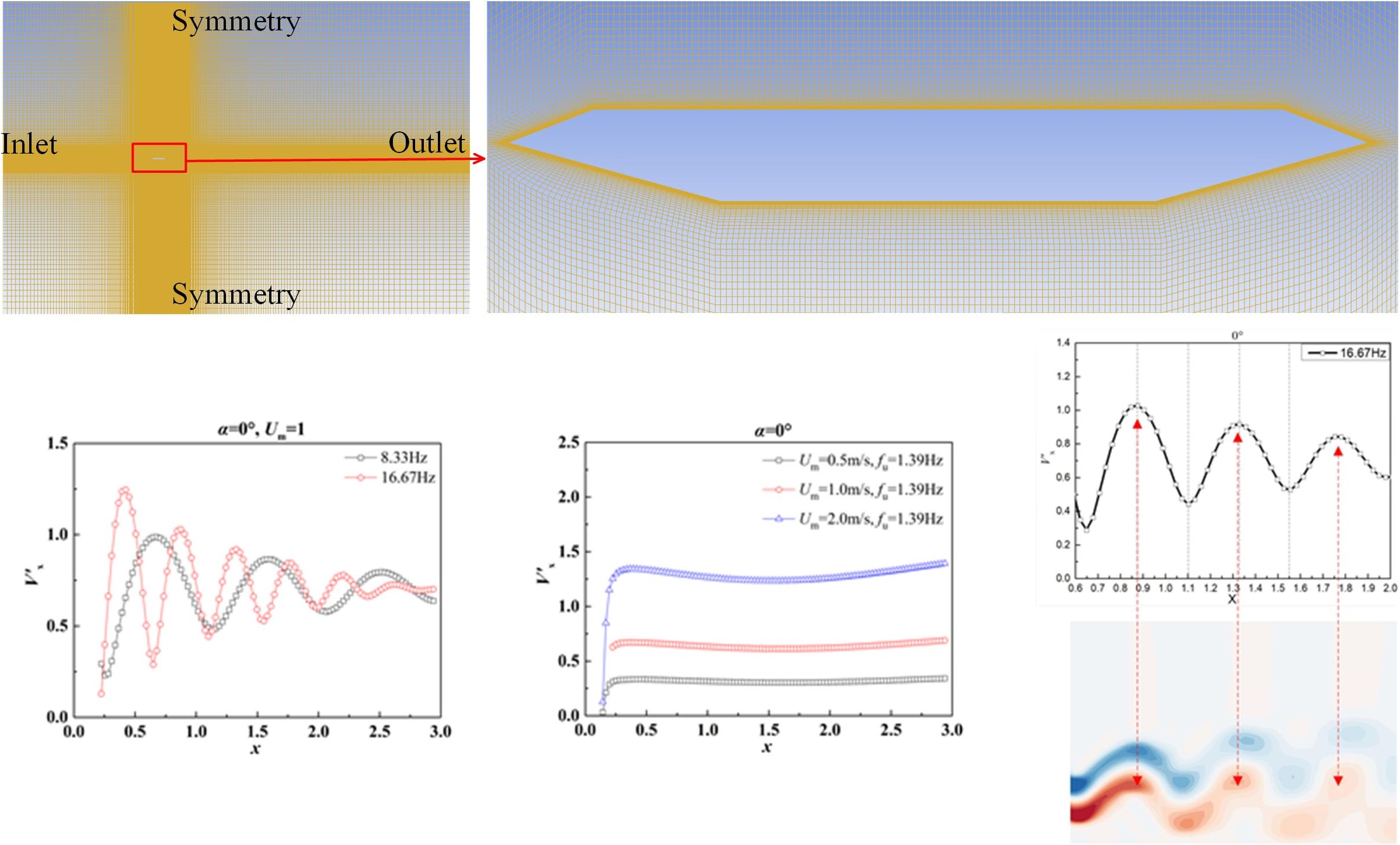#### Highlights

• The effects of various oscillation frequency and amplitude on the mean velocity, fluctuating velocity and vortex shedding distance of wake are investigated.
• The validity of the numerical simulation is verified by the active control wind tunnel experiment.
• The wake of a single-box girder in sinusoidal oscillating flow is studied by three-dimensional large-eddy simulations.

## 1. Introduction

A large number of long-span bridges have been built around the world in the past few decades. Box girder has become the preferred girder form for long-span bridges because of its good aerodynamic performance. However, the research on the wake of a single-box girder in a sinusoidal oscillation flow has not attracted enough attention.

In previous studies, most scholars focused on the wake of a circular cylinder. M. Braza et al.  and E. Konstantinidis et al.  investigated the wake characteristics of a circular cylinder. Wu Bo et al.  has investigated the near-wake characteristics of a rectangular cylinder in oscillating flow in recent years. However, the wake characteristics of single-box girder are quite different from the rectangular cylinder. There are still few studies on the wake of a single-box girder in the sinusoidal oscillating flow.

This paper investigated the effects of sinusoidal oscillations of various frequencies and amplitudes on the mean and fluctuating velocity, as well as vortex shedding distance of a single-box girder. It provides a reference for the aerodynamic characteristics of the wake flow of a single-box girder in the sinusoidal oscillation flow.

## 2. Numerical validation

### 2.1. Numerical method

Fig. 1 shows the geometric and detailed dimensions of the segmental model of the single-box girder. It is 0.28 m (B) wide and 0.03 m (D) high.

Fig. 1Geometry configuration of deck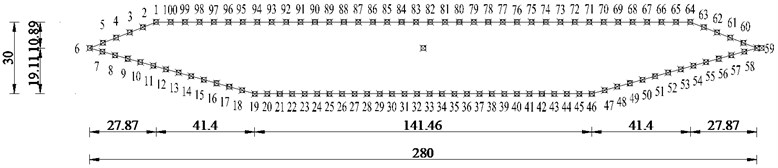Numerical simulations are carried out using three-dimensional large-eddy simulations (3D LES) with SGS model. The computational domain takes into consideration 10B in front, 20B behind, 10B above/under the surfaces to ensure domain-independence. Fig. 2 shows the computational domain and mesh distributions. At the inlet boundary, a sinusoidal velocity fluctuation is specified in the $x$-direction, which is defined as $U\left(t\right)=8+{U}_{m}\mathrm{s}\mathrm{i}\mathrm{n}\left(2\pi t{f}_{u}\right)$, where ${U}_{m}$ is the gust amplitude and ${f}_{u}$ is oscillating frequency.

Fig. 2Computational domain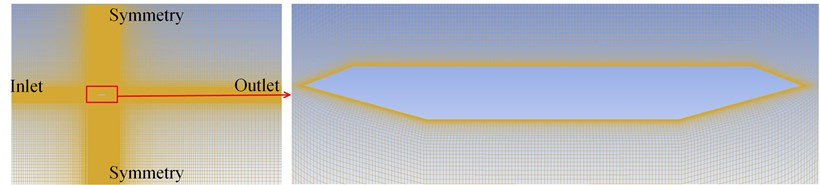### 2.2. Validation

As shown in Fig. 3, the numerical simulations are validated by the active control wind tunnel tests. The experimental tests are carried out in a multiple-fan active control wind tunnel at Tongji University, Shanghai, China. It can be observed from the figure that the value of CFD accurately reflects the variation trend of the mean pressure coefficients of the single-box girder. The value of CFD only deviates from the test value at the upper surface corners, and the error is still within an acceptable range. Moreover, it is obviously shown that the oscillating amplitude and frequency of sinusoidal flow have less impact on the mean pressure coefficients of the bridge section.

Fig. 3Comparisons of mean pressure coefficients obtained by experimental tests and numerical simulations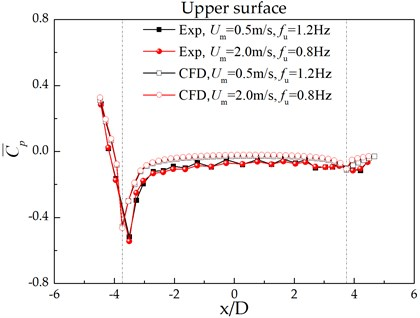## 3. Results discussion

### 3.1. Mean and fluctuating velocity

Fig. 4 shows the effect of oscillating amplitude and frequency on the mean velocity distributions around the wake in different sinusoidal oscillating flows. Due to the interference of the box girder, the mean velocity in the $x$-direction of the wake in the near-wake region (0.5) is significantly smaller than the mean incoming flow. As the flow distance increases, the mean velocity in the $x$-direction gradually increases to the mean incoming flow (8 m/s). The influences of oscillating frequency and amplitude on the changing trend can be ignored.

Fig. 4The distributions of mean velocity in the x-direction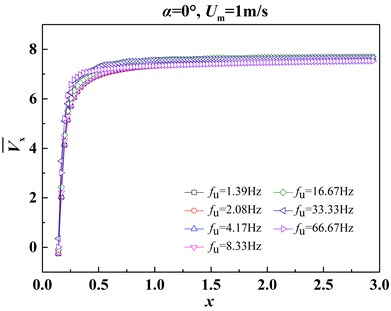a) The effect of frequency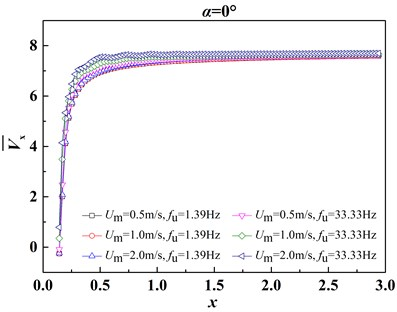b) The effect of amplitude

Fig. 5The distribution of fluctuating velocity in the x-direction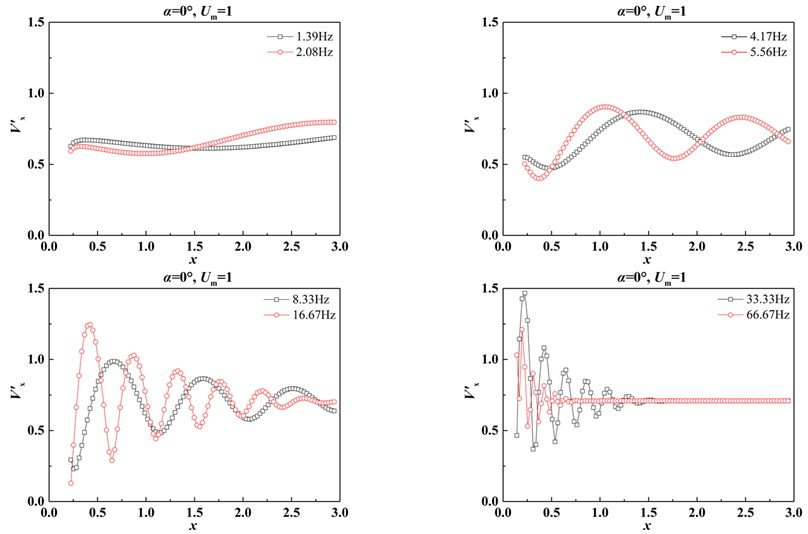a) The effect of frequency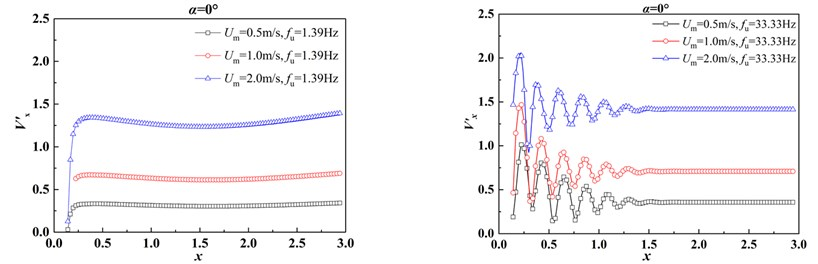b) The effect of amplitude

Fig. 5 shows the effect of oscillating amplitude and frequency on the fluctuating velocity distributions around the wake in different sinusoidal oscillating flows. As shown in Fig. 5(a), the fluctuating velocity is greatly influenced by the oscillating frequencies, its period decreases with the increase of oscillating frequency of the flow. This phenomenon is related to the vortex spacing of the wake, which will be discussed in detail later. With the increase of oscillating amplitude, the fluctuating velocity in wake increases obviously, however its period is not affected by oscillating amplitude.

### 3.2. Vortex shedding distance

In order to study the relationship between fluctuating velocity and vortex shedding, a typical case (${U}_{m}=$ 1 m/s, 16.67 Hz) is selected for investigation. As shown in Fig. 6, the upper part is the fluctuating velocity in the wake, and the lower part is the corresponding the wake vortex-shedding. It can be seen that the peak of the fluctuating velocity always corresponds to the center of the clockwise vortex. Conversely, the valley of the fluctuating velocity corresponds to the center of the counterclockwise vortex. Therefore, the shedding distance of the wake vortex can be calculated from the distance between the two peaks or two valleys of the fluctuating velocity curve.

Fig. 6Relationship between wake vortex spacing and fluctuating velocity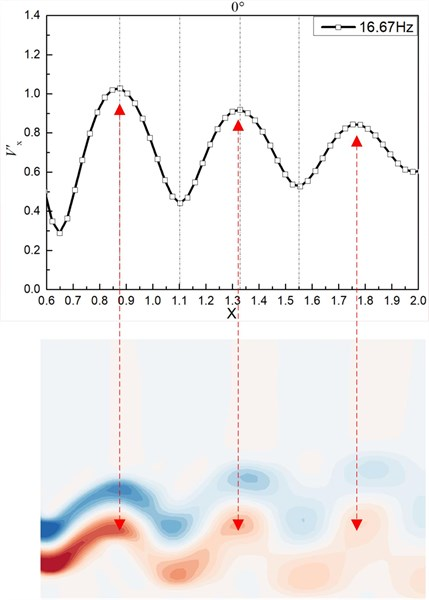Based on the above investigations, Fig. 7 shows the effects of oscillating amplitude and frequency on the vortex shedding distance in different sinusoidal oscillating flows. The vortex shedding distance decreases with the increase of the fluctuating frequency, but is not affected by the oscillation amplitude.

Fig. 7Vortex shedding distance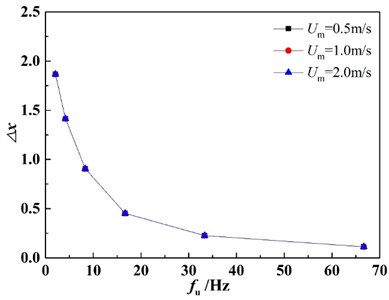## 4. Conclusion

Due to the active control wind tunnel cannot generate high-frequency sinusoidal oscillating flow, this paper mainly uses numerical simulation to investigate the wake of single-box girder in the sinusoidal oscillating flow. The conclusions are as follows:

1) The oscillating amplitude and frequency of sinusoidal flow have less impact on the mean pressure coefficients of the bridge section.

2) The distribution of mean wake flow of single-box girder in the effect of the various sinusoidal oscillating flow is basically same. As the oscillation amplitude increases, the fluctuating velocity in the wake increases significantly, but its period is not affected by the oscillation amplitude.

3) The wake vortex shedding distance gradually decreases with the increase of the inlet flow frequency, but regardless of the oscillation amplitude.

#### Acknowledgements

This study was funded by the Natural Science Foundation of Chongqing under grant number cstc2018jscx-msyb1299, the Science and Technology Research Program of Chongqing Municipal Education Commission under the grant number KJZD-K201802501, the Graduate Scientific Research and Innovation Foundation of Chongqing under the grant number CYB20028.